## Pedal-powered geologyWhat on earth am I doing here? Read on to find out. Many thanks to Simon Gage for the idea and to Graham Rose for the wonderful illustration.

In the previous post, we discovered that the kinetic energy of a drifting continent is of the same general magnitude as that of a moving bicycle and its rider – 1500 joules would be a typical figure.

I went on to calculate that, whereas it takes me only about 10 seconds to get my bike up to full speed, it would take me hundreds of years to get the continent up to its tiny full speed were I to put my shoulder against it and push (assuming that it was perfectly free to move). How can this be, when the amount of energy that I’m giving each of these objects is the same?

The problem is that when I push the continent, I am, effectively, in the wrong gear.

On a bike with gears, you’ve got a range of choices about how you power it: you can ride in a high gear, pedalling slowly but pushing hard on the pedals, or ride in a low gear, pedalling more quickly but pushing less hard on the pedals. There’s a simple tradeoff: if you want to pedal half as fast, you’ve got to push twice as hard for the same effect.

But there’s a limit to how hard you can push on the pedals, which means that if you move up too far up through the gears, there comes a point where you can no longer make up for the decreased pedalling rate by pushing harder on the pedals, and the power that you can supply to the bicycle falls.

Anyone who’s tried to accelerate a bicycle when they are in too high a gear will have experienced this problem, and it’s what I experience when I try to push the continent directly. Because the top speed of the continent is extremely low (about the speed of a growing fingernail), I’m necessarily pushing it very slowly as I accelerate it. This means that to give it energy at the rate that I want to (1500 joules in 10 seconds, like the bike) I would have to push it impossibly hard – the force needed is about the same as the weight of a 300-metre cube of solid rock.

Is there a way that we can put me into a lower gear, so that I can push with a force that suits me, over a longer distance, and still apply the very high force over a short distance to the continent?

Yes. Just as we’ve all used a screwdriver as a lever to get the lid of a tin of paint off, so I could use a lever to move the continent. Similarly to the bike gears, the lever allows me to exchange pushing hard over a small distance with pushing less hard over a longer distance. To do the job, the lever would need to be long enough to allow me to push, with all my might, through a distance of about 2.5 metres, with the short arm of the lever pushing the continent. We’d need an imaginary immoveable place for me to stand, and we could use the edge of the neighbouring continent as the pivot (just as we use the rim of a paint tin as the pivot). The catch is the length of the lever: if the short arm was 1 metre long, the long arm would be about 1.5 million kilometres long.

Simon Gage of Edinburgh International Science Festival suggested a more compact arrangement: a bicycle with an extremely low gear ratio, with the front wheel immobilised on the neighbouring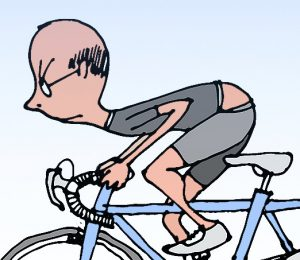continent (assumed immoveable), and the back wheel resting on the continent we’re trying to accelerate. A transmission giving 17 successive 4:1 speed reductions would do the job nicely. Ten seconds of hard pedalling would get the continent up to full speed. To me on the saddle, it shouldn’t feel any different to accelerating my bike away from the lights.

A wee caveat. This is a thought experiment, and we’ve swept some fairly significant engineering issues under the carpet. The rearmost parts of the power train would be moving at speeds that are literally geological, so in reality it would take me years of pedalling to take all of the slack and stretch out of the system. These parts would also be transmitting mountainous forces, and so they’d need to be supernaturally strong. There will be frictional losses. And then there’s the issue of transmitting a gigantic force to the continent through the contact of a bike tyre on the ground.

### The calculations

What force is required to accelerate the Eurasian plate to top speed in 10 seconds?

The top speed of the plate is 3.2 × 10-10 ms-1. If I accelerate it uniformly, its average speed will be half of this, and so in the 10 seconds over which I hope to accelerate it, it will travel 1.6 × 10-9 m.

Now W = fd

where W is the work that I do on the plate (ie the kinetic energy that I give it), f is the force that I apply to it, and d is the distance through which I push the plate. Rearranging gives us

f = W/d

We know W from the previous post (it’s 1500 joules) and we’ve just calculated d. Thus f works out at about 9.4 × 1011 newtons.

For comparison, a 300-metre cube of rock of density 2700 kg m-3 will have a weight of (300 m)3 × 2700 kg m-3 × 9.81 m s-2 = 7 × 1011 newtons roughly.

#### The lever

When a lever is used to amplify a force, the ratio of the lengths of the arms of the lever needs to be the same as the ratio of the two forces. Suppose that I can push with a force equal to my own body weight, about 600 newtons. If I’m to use a lever to amplify my push of 600 N to a force of 9.4 × 1011 N, the ratio of the lengths of the arms needs to be (9.4 × 1011)/600, or roughly 1.5 × 109. So if the short arm of the lever is 1 metre long, the long arm needs to be about 1.5 × 109 metres long, which is 1.5 million kilometres. For comparison, the Moon is about 400,000 kilometres away.

To do 1500 joules of work with a force of 600 N, I’d need to push over a distance of 2.5 metres (because 600 × 2.5 = 1500).

#### The bicycle gearing

I estimated that it takes me 15 pedal revolutions to get my bike up to full speed. Knowing the length of the pedal cranks, I know the total distance that I have pushed the pedals through, and I know how much work I have done on the bicycle – 1500 joules. (I’m ignoring energy losses here, because they are small at low speeds on a bike and the calculation is highly approximate anyway). Using work done = force × distance, this gives an average force on the pedals of about 94 newtons.

The 17 stages of 4:1 reduction mean that the back wheel is rotating 417 = 1.7 × 1010 times slower than I’m pedalling. The pedalling force is amplified in the same ratio, to give a force on the teeth of the rearmost gear of 1.6 × 1012 newtons. We now have to allow for the fact that the radius of the rear wheel is about twice the length of the pedal crank. This roughly halves the force available at the rim of the rear wheel, giving a force of about 8 × 1011 newtons, which is close to what we need.

## The kinetic energy of a drifting tectonic plate…

…is broadly similar to the kinetic energy of me and my bike as I pedal along.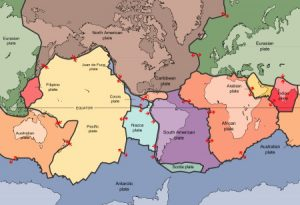Map of tectonic plates (United States Geological Survey) http://pubs.usgs.gov/publications/text/slabs.html

According the the theory of plate tectonics, the outer layer of the Earth is divided into a number of separate plates, which very slowly drift around, opening and closing oceans, causing earthquakes, and thrusting up mountain ranges.

A moving body has energy by virtue of its motion: kinetic energy. Kinetic energy is proportional to a body’s mass and to the square of its speed.

Now tectonic plates move extremely slowly: the usual comparison is with a growing fingernail. But they are also extremely heavy: tens of millions of square kilometres in area, over 100 km thick, and made of rock. I wondered how the minute speed and colossal mass play out against each other: what’s the kinetic energy of a drifting tectonic plate?

There are so many variables, that vary such a lot, that this calculation is going to be extremely approximate. But the answer is delightfully small: the kinetic energy of the tectonic plate on which I live, as observed from one of the plates next door, is about the same as the kinetic energy of me and my bike when I’m going at a reasonable pace: about 1500 joules.Me struggling up one of the many steep roads in north-west Scotland. Here, the kinetic energy of me and my bike is much less than the kinetic energy of a drifting tectonic plate. In fact the speed of me and my bike is probably much less than that of a drifting tectonic plate ;-).

This is a fun calculation to do, but we shouldn’t get carried away thinking about the kinetic energy of tectonic plates. Plates are driven by huge forces, and their motion is resisted by equally large forces. The mechanical work done by and against these forces will dominate a plate’s energy budget in comparison to its kinetic energy.

But the calculation does provoke an interesting thought about forces and motion. I can get my bike up to full speed in, say, 10 seconds. If the Eurasian plate were as free to move as my bike, and I were to put my shoulder against it and shove as hard as I could, it would take me about 500 years to get it up to its (very tiny) full speed.

In both cases, I’m giving the moving object roughly 1500 joules of kinetic energy. How come I can give that energy to my bike in a few seconds, but to give it to the plate would take me centuries?

I’ll return to that thought in a later post.

#### The calculation

Depending on how you count them, there are 6-7 major tectonic plates, 10 minor plates, and many more microplates. The plates vary hugely in size, from the giant Pacific Plate with an area of 100 million km2, to the dinky New Hebridean plate, which is a hundred times smaller. The microplates are smaller still. Plates also vary a lot in speed: 10-40 mm is typical.

I’m going to be parochial, and choose the Eurasian plate for this calculation.

Let’s call the area of the plate a and its mean thickness t. Its volume is then given by at, and if its mean density is ρ, then its mass m is ρat.

A body of mass m moving at a speed v has kinetic energy ½mv2. So our plate will have kinetic energy ½ρatv2.

The area of the Eurasian plate is 67,800,000 km2 or 6.78 × 1013 m2, and its speed relative to the African plate is (the only speed I have) is given as 7-14 mm per year. We’ll use 10 mm per year, which is 3.2 × 10-10 ms-1. The thickness of tectonic plates in general varies roughly in the range 100-200 km depending upon whether we are talking about oceanic or continental lithosphere; let’s call it 150 km or 1.5× 105 m. The density of lithospheric material varies in the range 2700-2900 kg m-3; we’ll use 2800 kg m-3.

Putting all of these numbers into our formula for kinetic energy, we get a value of 1500 joules (to 2 significant figures, which the precision of the input data certainly doesn’t warrant).

Now for me and my bike. I weigh about 57 kg, my bike is probably about 10 kg. Suppose I’m riding at 15 mph, which is 6.7 ms-1. My kinetic energy is almost exactly…

…1500 joules!

The closeness of these two values is unmitigated luck*, and we shouldn’t be  seduced by the coincidence. Just varying the speed of the plate in the range 7-14 mm would cause a 4-fold change in kinetic energy, and there’s the variability in plate thickness and rock density to take into account as well. The choice of bike speed was arbitrary, I guessed the mass of the bike, and I’ve since realised that I didn’t account for the fact that the wheels of my bike rotate as well as translate.

However, what we can say is that the kinetic energy of a drifting continent is definitely on a human scale, which leads to a new question:

Suppose the Eurasian plate were as free to move as my bicycle, and that I put my shoulder against it and shoved, how long would it take me to get it up to speed?

From the figures above, the mass of the plate is 2.85 × 1022 kg. If I can push with a force equal to my own weight (about 560 newtons) then by Newton’s 2nd Law I can give it an acceleration of about 1.96 × 10-20 ms-2. Rearranging the equation of motion v = at, where v is the final speed, a is the acceleration, and t is the time, then t = v/a. Inserting the values for v and a, we get t = 1.6 × 1010 seconds, or about 500 years.

* I didn’t tweak my assumptions: what you see above really is the very first version of the calculation!

## If I suddenly became weightless, what would happen to me?

Suppose that you were standing perfectly still, and gravity suddenly stopped operating on your body. What would happen? Nothing much, you might think, apart from a queasy feeling of weightlessness. After all, an object won’t start to move unless a force acts on it, and no force is acting on your body.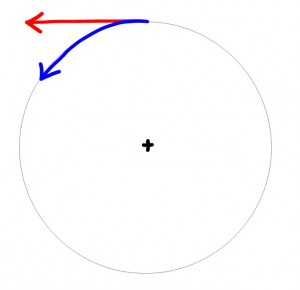Diagram of the Earth, looking straight down on the north pole.

However, when you stand “still”, in fact you’re travelling in a very large circle at rather high speed, as the Earth turns on its axis and carries you with it. Newton’s 1st Law tells us that things travel in straight lines unless a sideways force acts upon them. The force that keeps tugging you to make you travel in a circle rather than in a straight line is gravity. This means that the moment gravity stops acting on you, you’ll start moving along a straight line (the red line in the diagram) while the ground continues to move in a circular path underneath you (the blue line).

The consequence is that you’ll lose contact with the Earth and float upwards, serenely or otherwise. At least, that’s what it will look like to earthbound observers. But what’s really happening is that the ground is accelerating downwards away from you as it moves on its curved path. Try to remember that as you watch your footprints receding beneath you.

I wondered how fast this would all happen. The answer is: remarkably quickly. I give the geometry later, for those who are interested, but here are some example results for a person standing in Edinburgh, on a latitude of 56° N. Just for now, we’ll pretend that there isn’t any air.

• After 1 second your feet will be 5 millimetres off the ground.
• After 10 seconds you’ll be 53 centimetres off the ground.
• After a minute, you’ll be 19 metres up
• After an hour you’ll be at an altitude of over 68 km (though, being half frozen to death by now, you may be losing interest).

The lower your latitude, the quicker your ascent. At the equator, you’ll rise about three times as fast as in Edinburgh, and at the poles, you won’t lose contact with the ground at all.

Why on earth should I be interested in a situation that is contrived and physically impossible? It’s because it brings home the fact that each of us is constantly moving along a curved path as the Earth rotates. On the equator, it takes only 10 seconds for our trajectory to deviate from a straight line by 1.7 metres (during which time we’ve travelled 465 metres).

Why did I pretend that there isn’t any air? Because the presence of air muddies the waters by adding another force: the upward force of our buoyancy in the air.  At low altitudes this force isn’t negligible: it slightly more than doubles the first three figures above. As you rise further and the air gets thinner, it matters less and less. I left it out because I wanted to make the effect of the Earth’s rotation clear.

The question that I’ve just answered is a trimmed-down version of a question that my friend Malcolm and I occupied ourselves with once when we were on a rather long and boring tramp along a glen at the end of a camping trip in the Cairngorms in Scotland. The question we asked then was: what would we observe if gravity suddenly stopped operating altogether? I may return to that subject in a future post.

#### The geometry

Let the centre of the Earth be at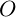, the origin, and let the Earth’s radius beand its angular velocity about its own axis be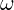. You are standing at latitude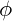and are therefore a distance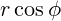from the Earth’s axis. Your linear velocity as you stand still on the rotating Earth will be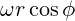, tangential to the Earth’s surface.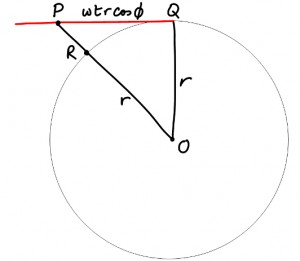Suppose that gravity stops acting on you at time zero, when you are at point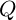. With no gravity acting on you, will now travel in a straight line tangential to the Earth’s surface. The diagram shows the situation from a suitable vantage point, looking sideways on to your direction of travel. We are not looking down on the north pole.

After a time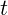, you will have travelled a distance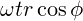, to point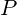.

Your altitude is the distance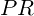, where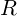is the point on the Earth’s surface for whichis directly overhead.lies on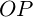, the line fromto the centre of the Earth.  The length ofis given directly by Pythagoras’ Theorem in triangle: it’s. As, the altitude of pointis. So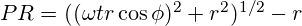All we need now is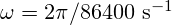, because the Earth does one full rotation in 86400 seconds, and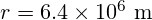, because that’s how big the Earth is. We can now chooseand calculatefor any value of.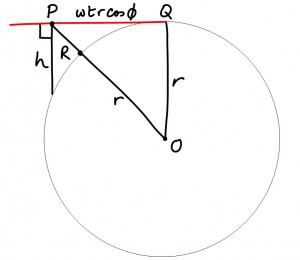We can check this answer in two ways. Firstly, we can use the very useful intersecting chords theorem to calculate the distance marked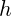in the diagram on the right. For small values of, where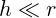, thenshould be approximately equal to the your altitude. For values of t of 1, 10, or 60 seconds,andagree to 4 significant figures. As we expect, asincreases, the agreement gets less good:anddiffer by about 2% after 1 hour.

The second check is to differentiate the expression fortwice with respect to time. The first differentation gives us an expression for the rate of change ofwith respect to time, that is, your rate of gain of altitude: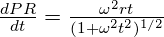(Note: to keep things clear, I’ve omitted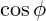, which merely accompanieseverywhere and doesn’t change the conclusions.) Where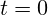, and your motion is purely tangential, this expression for your speed away from the ground should be zero, and whereis very large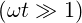, and your motion is purely radial, your speed away from the ground should be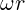. Both are true.

The second differentiation gives us an expression for your upward radial acceleration: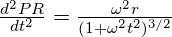As it’s not you accelerating, but the ground that is accelerating away from you as it continues on its circular path, this expression for your upwards acceleration should, whereand your path is tangential to the surface, become the same as that for the centripetal acceleration of the ground,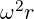, which it does. In addition, when, and your path is almost radial, the expression for your acceleration should approach zero, which it does.